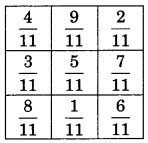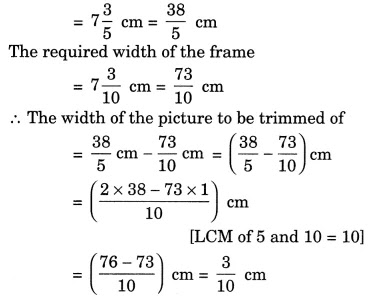NCERT Solutions for Class 7 Maths Chapter 2 Fractions and Decimals Ex 2.1

# NCERT Solutions for Class 7 Maths Chapter 2 Fractions and Decimals Ex 2.1

## NCERT Solutions for Class 7 Maths Chapter 2 Fractions and Decimals Ex 2.1

NCERT Solutions for Class 7 Maths Chapter 2 Fractions and Decimals Ex 2.1 are the part of NCERT Solutions for Class 7 Maths. Here you can find the NCERT Solutions for Class 7 Maths Chapter 2 Fractions and Decimals Ex 2.1.

Solution:

### Ex 2.1 Class 7 Maths Question 2.

Arrange the following in descending order:
(i) 2/9, 2/3, 8/21          (ii) 1/5, 3/7, 7/10

Solution:

### Ex 2.1 Class 7 Maths Question 3.

In a “magic square”, the sum of number in each row, in each column and along the diagonals is the same. Is this a magic square?Solution:

Since, the sum of the fraction in each row, each column and each diagonal is the same, i.e., 15/11.
Hence, it is a magic square.

### Ex 2.1 Class 7 Maths Question 4.

A rectangular sheet of paper is 121/2 cm long and 102/3 cm wide. Find its perimeter.

Solution:

### Ex 2.1 Class 7 Maths Question 5.

Find the perimeter of (i) ∆ABE (ii) the rectangle BCDE in this figure. Whose perimeter is greater?

Solution:
(i) Perimeter of ∆ABE

Thus, the perimeter of ∆ABE is greater than the perimeter of the rectangle BCDE.

### Ex 2.1 Class 7 Maths Question 6.

Salil wants to put a picture in a frame. The picture is 73/5 cm wide. To fit in the frame, the picture cannot be more than 73/10 cm wide. How much should the picture be trimmed?

Solution:
The width of the pictureHence, the required width of the picture to be trimmed is 3/10 cm.

### Ex 2.1 Class 7 Maths Question 7.

Ritu ate 3/5 part of an apple and the remaining apple was eaten by her brother Somu. How much part of the apple did Somu eat? Who had the larger share? By how much?

Solution:
Let the whole part of the apple be 1.

Thus, Ritu ate more share by 1/5 part.

### Ex 2.1 Class 7 Maths Question 8.

Michael finished colouring a picture in 7/12 hour. Vaibhav finished colouring the same picture in 3/4 hour. Who worked longer? By what fraction was it longer?

Solution:
Time taken by Michael = 7/12 hour
Time taken by Vaibhav = 3/4 hour